# VBA Optional Parameter

Functions that are used in VBA, be they built in functions or custom functions, can contain arguments. Arguments are variable values that are passed to the function in order to run the calculation as required. If these arguments are not always needs to run the function, they are called optional arguments or optional parameters.

## Custom Functions

Consider the following custom function that we are going to use the calculate the price of a product:

``````Function CalculatePrice(Qty As Double, UnitPrice As Double, Optional Cur As String) As String
Dim Price As Double
Price = Qty * UnitPrice
If Cur = "" Then
CalculatePrice = "\$" & Format(Price, "#,##0.00")
Else
CalculatePrice = Cur & Format(Price, "#,##0.00")
End If
End Function``````

Now to use this function, we can pass the quantity and unit price to the function, and if we wish to do so, the currency we wish to return the result in.

For example:

``````Sub TextPrice()
MsgBox CalculatePrice(20, 15.8, "£")
End Sub``````

This would return the following message box: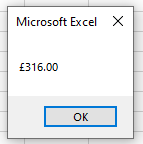However, if we were to run the following code:

``````Sub TextPrice()
MsgBox CalculatePrice(20, 15.8)
End Sub``````

Then the following message box would be returned.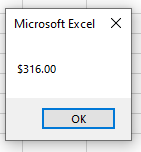This is due to the fact that in our function, the cur variable is optional. We have allowed for an empty string in our code so that if that variable does not contain a value, then a default value of “\$” is returned instead. We could also have left it out completely.

``````Function CalculatePrice(Qty As Double, UnitPrice As Double, Optional Cur As String) As String
Dim Price As Double
Price = Qty * UnitPrice
CalculatePrice = Cur & Format(Price, "#,##0.00")
End Function``````

The function above would then just return 316.00 if the cur optional variable was not populated when calling the function.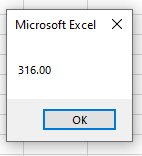If we always want the number to be formatted with the dollar sign, unless the user indicates a different currency, we can make use of the default value option instead of using an IF Statement.

``````Function CalculatePrice(Qty As Double, UnitPrice As Double, Optional Cur As String="\$") As String
Dim Price As Double
Price = Qty * UnitPrice
CalculatePrice = Cur & Format(Price, "#,##0.00")
End Function``````

Notice that in defining the optional parameter, we have put in a default value of “\$”.

This means that when the function is used, a user can leave out that parameter, and the dollar sign would be returned to the user, or, a user could put in a different currency symbol and this would then be returned.

For example:

``````Sub TextPrice()
MsgBox CalculatePrice(20, 15.8, "R")
End Sub``````

would return: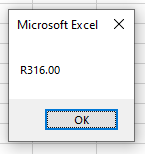whereas

``````Sub TextPrice()
MsgBox CalculatePrice(20, 15.8)
End Sub``````

would return:## Built in VBA Functions

Built in VBA functions often have optional parameters that we do not use when we use the functions.

For example if we were to use the MsgBox function, many of the parameters in this function are optional.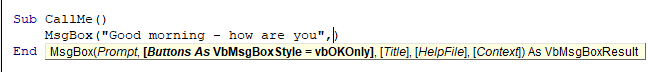In the syntax above, the only parameter that is required is the Prompt parameter.  As the other parameters are in square brackets, we know that these are optional parameters.  Some of these parameters may have a default value if not used like the Buttons parameter (vbOKOnly) while others can remain empty (Title, HelpFile, Context for example)

``````Sub CallMe()
MsgBox ("Good morning - how are you")
End Sub``````

If we run the above macro, this message box will appear: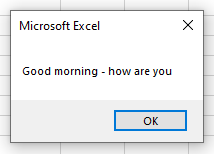However, if we amend the macro to this below:

``````Sub CallMe()
MsgBox "Good morning - how are you", vbQuestion, "HELLO USER!"
End Sub``````

Then a more customized box will appear: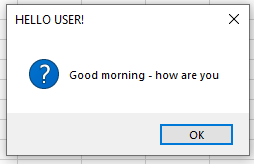Both macros will run but the second one enables us to customize the msgbox will a few more options!